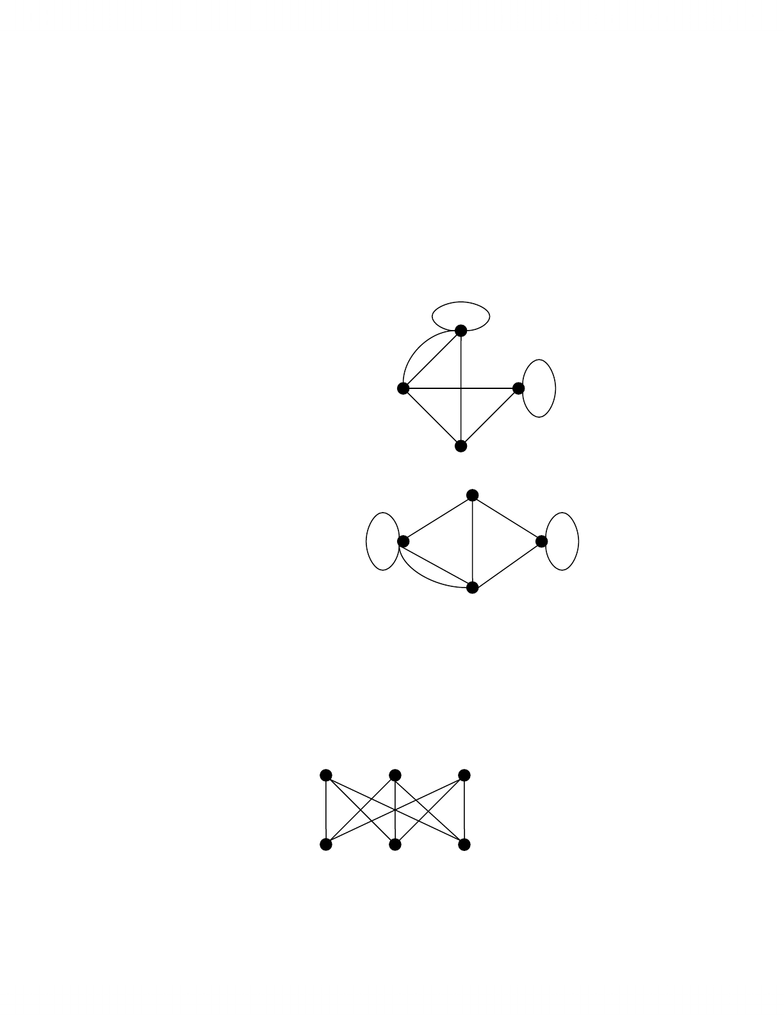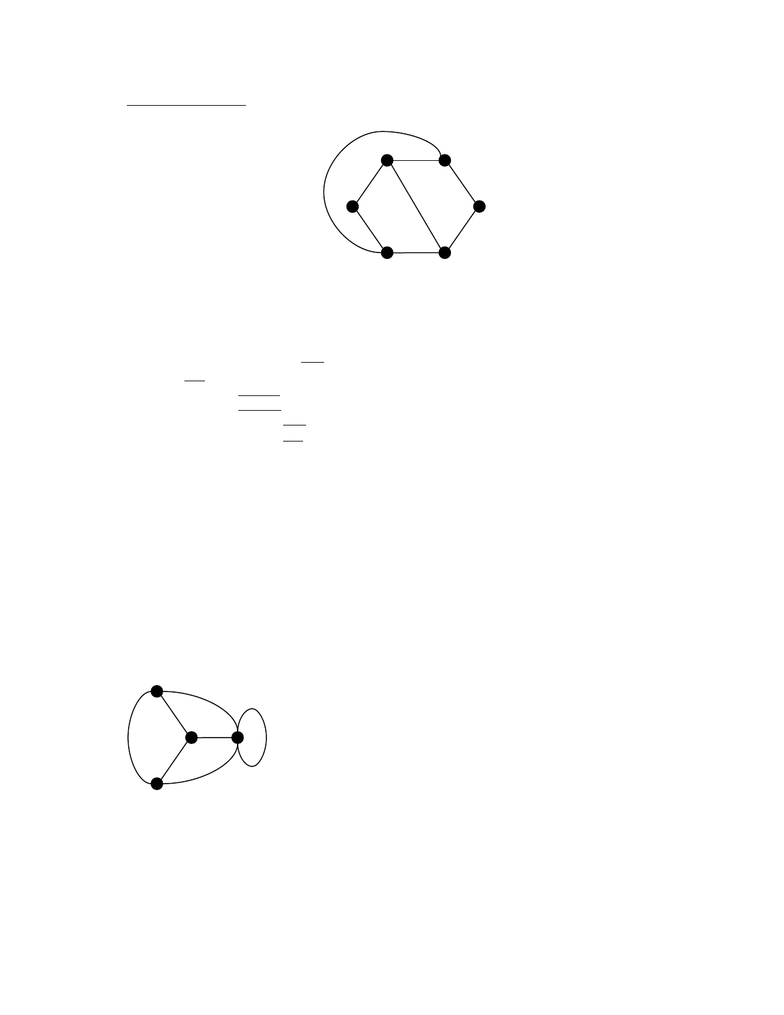# Summary notes

155 views32 pages
School
Department
Course
ProfessorMAT344H1.doc
Page 1 of 32
Graphs and Subgraphs
Definition: Graph
A graph G is a triple
(
)
(
)
(
)
G
GEGV
ψ
,, where
1)
(
)
GV is a finite set. The elements of
(
)
GV are called the vertices of G.
2)
(
)
GE is a finite set. The elements of
(
)
GE are called the edges.
3) G
ψ
is a function which associates to every edge of an unordered pair of not necessarily distinct vertices. G
ψ
is called the incidence function.
Example
Let
(
)
{
}
4321 ,,, vvvvGV=.
Let
(
)
{
}
87654321 ,,,,,,, eeeeeeeeGE=.
Let
(
)
111 vve
G=
ψ
,
(
)
232 vve
G=
ψ
,
(
)
223 vve
G=
ψ
,
(
)
314 vve
G=
ψ
,
(
)
325 vve
G=
ψ
,
(
)
146 vve
G=
ψ
,
(
)
427 vve
G=
ψ
,
(
)
438 vve
G=
ψ
.
The graph looks like:
Could have drawn it like this:
Definition: Planar
If a graph G can be drawn such that edges only intersect at their ends, then G is called planar.
Example
Consider
3,3
k. Prove that its not planar.
v1
v4
v2 v3
e1 e2
e3 e4 e5
e6 e7
e8
v5
v6
3,3
k
e9
v1
v4
v2
v3
v1
v4
v2 e1
e2
e3
e4
e5
e6
e7
e8
www.notesolution.com
Unlock document

This preview shows pages 1-3 of the document.
Unlock all 32 pages and 3 million more documents.MAT344H1.doc
Page 2 of 32
Cycle-Chord technique. Observe that
3,3
k contains a cycle which covers every vertex:
1258396624411
vevevevevevev .
There is nowhere to place
9
e!
Vocabulary
1) If
(
)
uve =
ψ
, then u and v are the ends of e.
2) e is said to join u and v.
3) u and v are said to be incident to the edge.
4) u and v are said to be adjacent.
5) If
v
u
=
, then e is said to be a loop.
6) If
v
u
, then e is said to be a link.
Definition: Simple
A graph is said to be simple if it has no loops and no two edges join the same pair of vertices.
VERTEX DEGREES
Definition: Vertex Degrees
The degree of a vertex v of a graph G, denoted
(
)
vdG, is defined to be the number of edges that end at the
vertex (loops count twice).
Example
Fact
(
)
( )
=
GVv
Gvd
ε
2, where
ε
is the number of edges.
Proof:
To begin, cut each edge into two pieces. Count the resulting half-edges in two different ways.
3
3
3 5
v1
v4
v2
v3
e1
e2
e3
e4
e5
e6
e7
e8
v5
v6
www.notesolution.com
Unlock document

This preview shows pages 1-3 of the document.
Unlock all 32 pages and 3 million more documents.MAT344H1.doc
Page 3 of 32
Count 1: Label every half edge by the vertex it ends at. The number of edges labeled with some vertex v is
clearly
(
)
vdG. So the number of half-edges is
(
)
( )
GVv
Gvd.
Count 2: There are clearly twice as many half-edges as edges.
Fact
In a graph, the number of vertices with odd degree is even.
Proof:
Let
(
)
(
)
GVGVeven be the set of vertices of G with even degrees. Similarly define
(
)
(
)
GVGVodd .
(
)
( )
(
)
( )
(
)
( )
+==
oddeven
2
GVv
G
GVv
G
GVv
Gvdvdvd
ε
, so
(
)
( )
oddGVv
Gvd must be even, so the number of vertices
with odd degree must be even.
Problem
1) In a group of 8 persons, is it possible that everyone knows exactly 3 others? Yes.
2) In a group of 7 persons, is it possible that everyone knows exactly 3 others? No.
APPLICATION: THE MOUNTAIN CLIMBERS PUZZLE
Consider 2 mountaineers approaching a mountain range from opposite sides. Is it possible for the climbers to
always be at the same height and reach the summit?
Theorem
Two mountaineers can indeed clime in the required fashion to the summit of any mountain range.
Proof:
Construct a graph (the ascent graph) with vertices corresponding to configurations of the climbers where:
1) The two climbers are at the same height.
2) There is one climber on each side of the summit.
3) At least one of the climbers is at a local maxima or minima.
Join the vertices by an edge when the climbers can move between the corresponding configurations by strictly
ascending or strictly descending together.
We must show that no matter for what mountain range, the corresponding ascent graph G has a path from the
initial configuration
(
)
ZA, to the summit
(
)
MM ,.
Well assume no such path exists and produce a contradiction. The proof follows from two observations on G.
Observation 1: G has exactly 2 vertices of degree 1 (namely, the initial configuration and the summit), and the
rest are either degree 2 or 4 (2 when one is on a local max/min, 4 when both are on local max/min at the same
height).
Observation 2: Let
( )
(
)
GVV
ZA
, denote the set of vertices that can be reached by a path from
(
)
ZA,. Note
that the summit
(
)
( )
ZA
VMM ,
,. It is easy to see that there are no edges in G with one end in
( )
ZA
V, and one
end in
(
)
( )
ZA
VGV,
\.
www.notesolution.com
Unlock document

This preview shows pages 1-3 of the document.
Unlock all 32 pages and 3 million more documents.